# Pediatrician

Pediatrician this month of 20 working days takes 8 days holidays. What is the probability that on Monday will be at work?

Correct result:

p =  0.6

#### Solution:

$p=1-\frac{8}{20}=0.6$We would be pleased if you find an error in the word problem, spelling mistakes, or inaccuracies and send it to us. Thank you!Tips to related online calculators
Need help calculate sum, simplify or multiply fractions? Try our fraction calculator.
Check out our ratio calculator.
Do you want to convert time units like minutes to seconds?
Would you like to compute count of combinations?

## Next similar math problems:

• Ratio of countsThere are 15 boys and 13 girls in the class. What are the ratio of boys and girls?
• The machineThe machine was in operation for 9 tenths (in a fraction) of eight hours of working time. How long has he been running?
• Masons1 mason casts 30.8 meters square in 8 hours. How long casts 4 masons 178 meters square?After the history test, Michaella discovered that the ratio of her correct and incorrect answers is 5: 3. How many correct answers did Michaella have in the test, if she had 6 incorrect answers?
• KingKing had four sons. First inherit 1/2, second 1/4 , third 1/5 of property. What part of the property was left to the last of the brothers?
• AgesFather is 36 years old, his daughter is 4 years old. Write down the ratio of the age of father and daughter. In what ratio will the ages of father and daughter after 4 years?
• Age ratioJanko is 14 years old. The age ratio of Janka and Zuzka is 2: 3. What was the ratio seven years ago?
• Pizza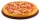Three siblings ordered one pizza. Miška ate a quarter of the whole pizza. Lenka ate a third of the rest and Patrik ate half of what Lenka had left. They had the rest packed up. How much of the pizza did they pack? Write the result as a fraction.
• DonutsFind how many donuts each student will receive if you share 126 donuts in a ratio of 1:5:8
• Screws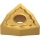The box contains 8 iron, 6 brass, and 4 titanium screws. What is the probability that the randomly selected screw will not be brass?
• Cups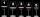We have three cups. In the cups we had fluid and boredom we started to shed. 1 We shed one-third of the fluid from the second glass into the first and third. 2 Then we shed one quarter cup of liquid from the first to the second and to the third. 3 Then we
• DivideDivide the number 72 in the ratio 7: 2 and calculate the ratio of the numbers found in this order and write down as decimal.
• Study results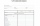There are 7 students in the class with excellent results, 6 praiseworthy, 5 good, 4 sufficient and 3 insufficient students. What is the probability that it will be a good student when summoned?
• Father and daughterFather is 36 years old, daughter is 20 years less. What will be the ratio between them when they are 10 years more?
• Brass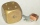Brass is an alloy of copper and zinc in a ratio of 3: 2. How many grams does a component that required 270 g of copper weigh?
• Salt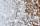1 t of sea water contains 25 kg of salt. How many tons of seawater must be evaporated to obtain 2 q of salt?
• Land areaA land area of Asia and Africa are in a 3: 2 ratio, the European and African are is 1:3. What are the proportions of Asia, Africa, and Europe?